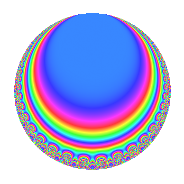Properties

 Label 128.4.b.eLevel 128 Weight 4 Character orbit 128.b Analytic conductor 7.552 Analytic rank 0 Dimension 4 CM No Inner twists 4

Related objects

Newspace parameters

 Level: $$N$$ = $$128 = 2^{7}$$ Weight: $$k$$ = $$4$$ Character orbit: $$[\chi]$$ = 128.b (of order $$2$$ and degree $$1$$)

Newform invariants

 Self dual: No Analytic conductor: $$7.55224448073$$ Analytic rank: $$0$$ Dimension: $$4$$ Coefficient field: $$\Q(\sqrt{2}, \sqrt{-5})$$ Coefficient ring: $$\Z[a_1, \ldots, a_{7}]$$ Coefficient ring index: $$2^{9}$$ Sato-Tate group: $\mathrm{SU}(2)[C_{2}]$

$q$-expansion

Coefficients of the $$q$$-expansion are expressed in terms of a basis $$1,\beta_1,\beta_2,\beta_3$$ for the coefficient ring described below. We also show the integral $$q$$-expansion of the trace form.

 $$f(q)$$ $$=$$ $$q$$ $$-\beta_{1} q^{3}$$ $$+ \beta_{3} q^{5}$$ $$+ \beta_{2} q^{7}$$ $$-13 q^{9}$$ $$+O(q^{10})$$ $$q$$ $$-\beta_{1} q^{3}$$ $$+ \beta_{3} q^{5}$$ $$+ \beta_{2} q^{7}$$ $$-13 q^{9}$$ $$+ 7 \beta_{1} q^{11}$$ $$-\beta_{3} q^{13}$$ $$+ 5 \beta_{2} q^{15}$$ $$+ 70 q^{17}$$ $$-13 \beta_{1} q^{19}$$ $$-8 \beta_{3} q^{21}$$ $$+ 7 \beta_{2} q^{23}$$ $$-195 q^{25}$$ $$-14 \beta_{1} q^{27}$$ $$+ 7 \beta_{3} q^{29}$$ $$+ 280 q^{33}$$ $$+ 64 \beta_{1} q^{35}$$ $$+ 21 \beta_{3} q^{37}$$ $$-5 \beta_{2} q^{39}$$ $$-182 q^{41}$$ $$-21 \beta_{1} q^{43}$$ $$-13 \beta_{3} q^{45}$$ $$-14 \beta_{2} q^{47}$$ $$+ 169 q^{49}$$ $$-70 \beta_{1} q^{51}$$ $$-7 \beta_{3} q^{53}$$ $$-35 \beta_{2} q^{55}$$ $$-520 q^{57}$$ $$-13 \beta_{1} q^{59}$$ $$-13 \beta_{3} q^{61}$$ $$-13 \beta_{2} q^{63}$$ $$+ 320 q^{65}$$ $$+ 35 \beta_{1} q^{67}$$ $$-56 \beta_{3} q^{69}$$ $$+ 5 \beta_{2} q^{71}$$ $$+ 910 q^{73}$$ $$+ 195 \beta_{1} q^{75}$$ $$+ 56 \beta_{3} q^{77}$$ $$-30 \beta_{2} q^{79}$$ $$-911 q^{81}$$ $$-113 \beta_{1} q^{83}$$ $$+ 70 \beta_{3} q^{85}$$ $$+ 35 \beta_{2} q^{87}$$ $$-546 q^{89}$$ $$-64 \beta_{1} q^{91}$$ $$+ 65 \beta_{2} q^{95}$$ $$-490 q^{97}$$ $$-91 \beta_{1} q^{99}$$ $$+O(q^{100})$$ $$\operatorname{Tr}(f)(q)$$ $$=$$ $$4q$$ $$\mathstrut -\mathstrut 52q^{9}$$ $$\mathstrut +\mathstrut O(q^{10})$$ $$4q$$ $$\mathstrut -\mathstrut 52q^{9}$$ $$\mathstrut +\mathstrut 280q^{17}$$ $$\mathstrut -\mathstrut 780q^{25}$$ $$\mathstrut +\mathstrut 1120q^{33}$$ $$\mathstrut -\mathstrut 728q^{41}$$ $$\mathstrut +\mathstrut 676q^{49}$$ $$\mathstrut -\mathstrut 2080q^{57}$$ $$\mathstrut +\mathstrut 1280q^{65}$$ $$\mathstrut +\mathstrut 3640q^{73}$$ $$\mathstrut -\mathstrut 3644q^{81}$$ $$\mathstrut -\mathstrut 2184q^{89}$$ $$\mathstrut -\mathstrut 1960q^{97}$$ $$\mathstrut +\mathstrut O(q^{100})$$

Basis of coefficient ring in terms of a root $$\nu$$ of $$x^{4}\mathstrut +\mathstrut$$ $$4$$ $$x^{2}\mathstrut +\mathstrut$$ $$9$$:

 $$\beta_{0}$$ $$=$$ $$1$$ $$\beta_{1}$$ $$=$$ $$($$$$2 \nu^{3} + 14 \nu$$$$)/3$$ $$\beta_{2}$$ $$=$$ $$($$$$-16 \nu^{3} - 16 \nu$$$$)/3$$ $$\beta_{3}$$ $$=$$ $$8 \nu^{2} + 16$$
 $$1$$ $$=$$ $$\beta_0$$ $$\nu$$ $$=$$ $$($$$$\beta_{2}\mathstrut +\mathstrut$$ $$8$$ $$\beta_{1}$$$$)/32$$ $$\nu^{2}$$ $$=$$ $$($$$$\beta_{3}\mathstrut -\mathstrut$$ $$16$$$$)/8$$ $$\nu^{3}$$ $$=$$ $$($$$$-$$$$7$$ $$\beta_{2}\mathstrut -\mathstrut$$ $$8$$ $$\beta_{1}$$$$)/32$$

Character Values

We give the values of $$\chi$$ on generators for $$\left(\mathbb{Z}/128\mathbb{Z}\right)^\times$$.

 $$n$$ $$5$$ $$127$$ $$\chi(n)$$ $$-1$$ $$1$$

Embeddings

For each embedding $$\iota_m$$ of the coefficient field, the values $$\iota_m(a_n)$$ are shown below.

For more information on an embedded modular form you can click on its label.

Label $$\iota_m(\nu)$$ $$a_{2}$$ $$a_{3}$$ $$a_{4}$$ $$a_{5}$$ $$a_{6}$$ $$a_{7}$$ $$a_{8}$$ $$a_{9}$$ $$a_{10}$$
65.1
 −0.707107 + 1.58114i 0.707107 + 1.58114i 0.707107 − 1.58114i −0.707107 − 1.58114i
0 6.32456i 0 17.8885i 0 −22.6274 0 −13.0000 0
65.2 0 6.32456i 0 17.8885i 0 22.6274 0 −13.0000 0
65.3 0 6.32456i 0 17.8885i 0 22.6274 0 −13.0000 0
65.4 0 6.32456i 0 17.8885i 0 −22.6274 0 −13.0000 0
 $$n$$: e.g. 2-40 or 990-1000 Significant digits: Format: Complex embeddings Normalized embeddings Satake parameters Satake angles

Inner twists

Char. orbit Parity Mult. Self Twist Proved
1.a Even 1 trivial yes
4.b Odd 1 yes
8.b Even 1 yes
8.d Odd 1 yes

Hecke kernels

This newform can be constructed as the intersection of the kernels of the following linear operators acting on $$S_{4}^{\mathrm{new}}(128, [\chi])$$:

 $$T_{3}^{2}$$ $$\mathstrut +\mathstrut 40$$ $$T_{7}^{2}$$ $$\mathstrut -\mathstrut 512$$